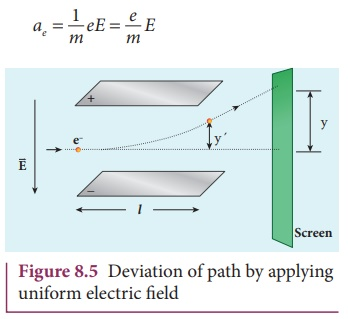Home | | Physics 12th Std | Determination of specific charge (e/m) of an electron - Thomson’s experiment

# Determination of specific charge (e/m) of an electron - Thomson’s experiment

(i) Determination of velocity of cathode rays (ii) Determination of specific charge (iii) Deflection of charge only due to uniform electric field (iv) Deflection of charge only due to uniform magnetic field

Determination of specific charge (e/m) of an electron - Thomson’s experiment

Thomson’s experiment is considered as one among the landmark experiments for the birth of modern physics. In 1887, J. J. Thomson made remarkable improvement in the scope of study of gases in discharge tubes. In the presence of electric and magnetic fields, the cathode rays are deflected. By the variation of electric and magnetic fields, mass normalized charge or the specific charge (charge per unit mass) of the cathode rays is measured.The arrangement of J. J. Thomson’s experiment is shown in Figure 8.3. A highly evacuated discharge tube is used and cathode rays (electron beam) produced at cathode are attracted towards anode disc A. Anode disc is made with pin hole in order to allow only a narrow beam of cathode rays. These cathode rays are now allowed to pass through the parallel metal plates, maintained at high voltage as shown in Figure 8.3. Further, this gas discharge tube is kept in between pole pieces of magnet such that both electric and magnetic fields are perpendicular to each other. When the cathode rays strike the screen, they produce scintillation and hence bright spot is observed. This is achieved by coating the screen with zinc sulphide.

## (i) Determination of velocity of cathode raysFor a fixed electric field between the plates, the magnetic field is adjusted such that the cathode rays (electron beam) strike at the original position O (Figure 8.3). This means that the magnitude of electric force is balanced by the magnitude of force due to magnetic field as shown in Figure 8.4. Let e be the charge of the cathode rays, then

eE =eBυ## (ii) Determination of specific charge

Since the cathode rays (electron beam) are accelerated from cathode to anode, the potential energy of the electron beam at the cathode is converted into kinetic energy of the electron beam at the anode. Let V be the potential difference between anode and cathode, then the potential energy is eV. Then from law of conservation of energy,Substituting the value of velocity from equation (8.1), we getSubstituting the values of E, B and V, the specific charge can be determined as

e/m = 1.7×1011 C kg−1

## (iii) Deflection of charge only due to uniform electric field

When the magnetic field is turned off, the deflection is only due to electric field. The deflection in vertical direction is due to the electric force.

Fe = eELet m be the mass of the electron and by applying Newton’s second law of motion, acceleration of the electron isSubstituting equation (8.4) in equation (8.3),Let y be the deviation produced from original position on the screen as shown in Figure 8.5. Let the initial upward velocity of cathode ray be u = 0 before entering the parallel electric plates. Let t be the time taken by the cathode rays to travel in electric field. Let l be the length of one of the plates, then the time taken isHence, the deflection of cathode rays is (note: u = 0 and ae = e/m E )Therefore, the deflection y on the screen is

y y y = C y

where C is proportionality constant which depends on the geometry of the discharge tube and substituting value in equation 8.6, we getRearranging equation (8.7) asSubstituting the values on RHS, the value of specific charge is calculated as e/m = 1.7 ×1011C kg−1

## (iv) Deflection of charge only due to uniform magnetic field

Suppose that the electric field is switched off and only the magnetic field is switched on. Now the deflection occurs only due to magnetic field. The force experienced by the electron in uniform magnetic field applied perpendicular to its path is

Fm = eυB

(in magnitude)

Since this force provides the centripetal force, the electron beam undergoes a semi- circular path. Therefore, we can equate Fm to centripetal force mυ2 / R.where v is the velocity of electron beam at the point where it enters the magnetic field and R is the radius of the circular path traversed by the electron beam.Further, substituting equation (8.1) in equation (8.10), we getBy knowing the values of electric field, magnetic field and the radius of circular path, the value of specific charge (e/m) can be calculated, which is also consistant with other two methods.

The specific charge is independent of (a) gas used (b) nature of the electrodes

Tags : Electric Discharge Through Gases | Physics , 12th Physics : UNIT 9 : Atomic and Nuclear Physics
Study Material, Lecturing Notes, Assignment, Reference, Wiki description explanation, brief detail
12th Physics : UNIT 9 : Atomic and Nuclear Physics : Determination of specific charge (e/m) of an electron - Thomson’s experiment | Electric Discharge Through Gases | Physics